Euclideanness is localization-closed

This article gives the statement, and possibly proof, of a commutative unital ring property (i.e., Euclidean ring) satisfying a commutative unital ring metaproperty (i.e., localization-closed property of commutative unital rings)
View all commutative unital ring metaproperty satisfactions | View all commutative unital ring metaproperty dissatisfactions |Get help on looking up metaproperty (dis)satisfactions for commutative unital ring properties
Get more facts about Euclidean ring|Get more facts about localization-closed property of commutative unital rings

Statement

Suppose$R$ is a Euclidean ring and$S$ is a multiplicatively closed subset of$R$ not containing any zero divisors (without loss of generality, we may assume that$S$ is a saturated multiplicatively closed subset. Let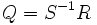$Q = S^{-1}R$ be the localization of$R$ at$S$. Then,$Q$ is also a Euclidean ring. Further, if$N$ is a Euclidean norm on$R$, we can define a new Euclidean norm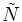$\tilde{N}$ on$Q$ as follows: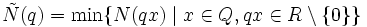$\tilde{N}(q) = \min \{ N(qx) \mid x \in Q, qx \in R \setminus \{ 0 \} \}$.

To see that this is well-defined, observe that any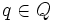$q \in Q$ can be expressed as$s^{-1}r$ for some$s \in S$,$r \in R$, and if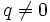$q \ne 0$,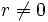$r \ne 0$. Thus,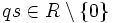$qs \in R \setminus \{ 0 \}$. Hence, the set on the right side is nonempty. A minimum over a nonempty well-ordered set is well-defined, so the expression is well-defined.

Note that doing this operation for$S = \{ 1 \}$ does not necessarily give back the same Euclidean norm as we started with. It gives back the same norm only if the original norm was multiplicatively monotone.

Proof

Fill this in later

==